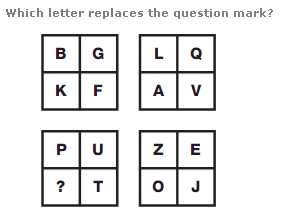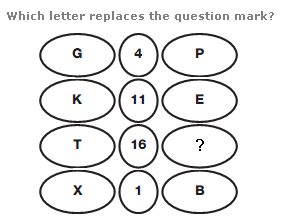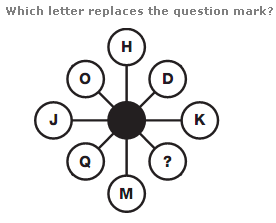# Puzzles - Missing letters puzzles

### Exercise :: Missing letters puzzlesAnswer : Y Explanation : Work in rows, across the 4 squares as a whole. Start at the top left and move to the right, then down one row and to the left etc in a snakes and ladders pattern. Letters advance through the alphabet in steps of 5 letters at a time.Answer : W Explanation : In each row, take the reverse alphabetical value of the left hand letter and the alphabetical value of the right hand letter and calculate the difference, putting this result in the central square.Answer : F Explanation : Taking pairs of letters at opposite sides of the diagram, the sum of their numerical values equals 21 each time.